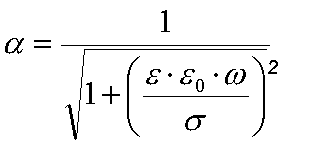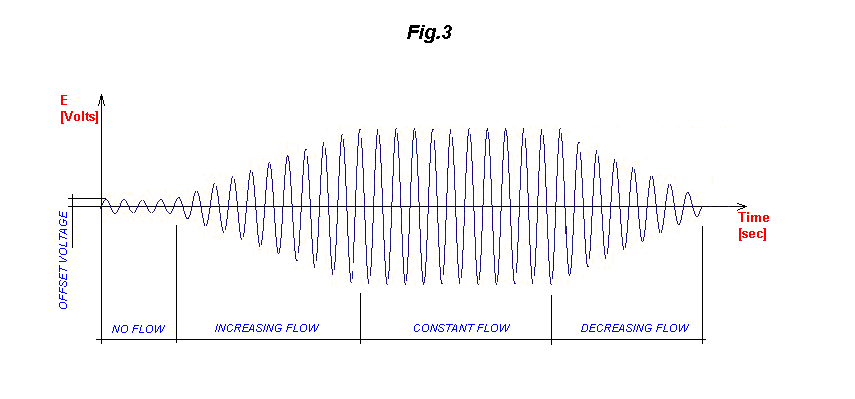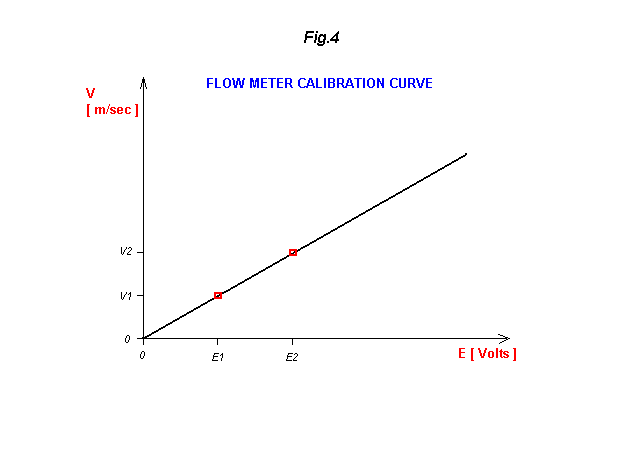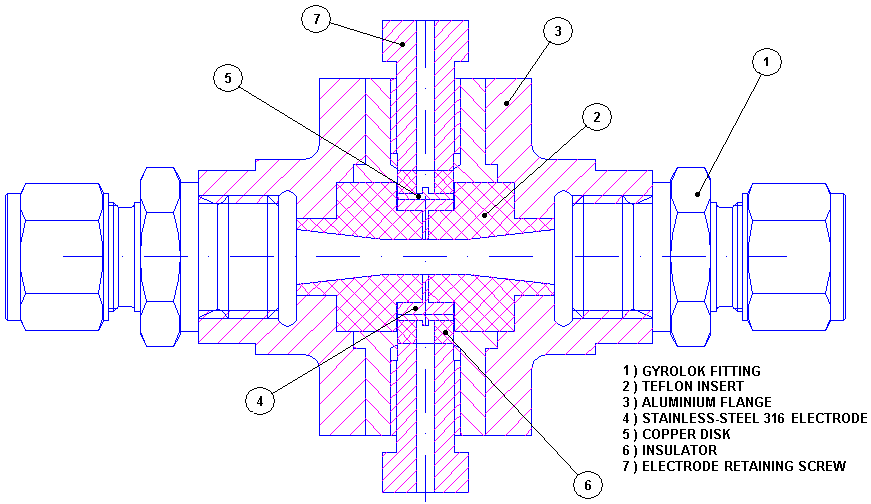Electromagnetic Flow Meter Tutorial

by
david.gagliardi@libero.it

 What is an Electromagnetic Flow Meter

An Electromagnetic Flow Meter is a device capable of measuring the mass flow of a fluid.
Unlike the common flow meter you can find on the market it has no moving parts, and for this reason it can be made to withstand any pressure (without leakage) and any fluid (corrosive and non corrosive).
This kind of flow meter use a magnet and two electrodes to peek the voltage that appear across the fluid moving in the magnetic field.

 How does it WorkThe Neumann Law (or Lenz Law) states that if a conductive wire is moving at right angle through a magnetic field, a voltage E [Volts] will appear at the end of the conductor (Fig.1):

E=B*L*V

were
B = Magnetic Induction [Weber/m2]
L = Length of the portion of the wire 'wetted' by the magnetic field [m]
V = Velocity of the wire [m/sec]

Now imagine you have a plastic tube with two electrodes on the diameter and Mercury flowing into it (fig.2).  A voltage will appear on the electrodes and it will beE=B*L*V

as in the previous example (L in this case is the inner diameter of the tube).
You can think of Mercury as tiny conductive wires next to each other : each wire, moving in the tube, will touch the two electrodes ,and thus you can measure their voltage.
An interesting fact is that if you reverse the flow, you still get a voltage but with reverse polarity (Fig.1).
Till now we have talked about a conductive fluid ,Mercury, but this stuff will also work with non conductive fluid ,provided that you use an alternating magnetic field.
Two physicists, Mittlemann and Cushing, in an unpublished work, stated that when using a non conductive fluid, if the frequency of the alternating magnetic field is v the voltage at the electrodes will be attenuated by a factor a so that:

E=B*L*V*a

were:ïo = Permittivity of Free Space [Farads / m]
s = Electrical Conductivity [MV / m]
v = Frequency [1 / sec]
ï and s depends on the substance used and can be found on a good chemistry book. ïo is a constant = 8.86E-12 [Farads / m].
If an alternating magnetic field is used, an alternating voltage will appear on the electrodes and its amplitude will be E.  That is, the output is an amplitude modulated wave.

 Measuring the flow
Measuring the flow present some difficulties.
A perfect axisimmetric construction cannot be achieved and thus some magnetic flux lines will 'wet' the connecting wires to the electrodes.  The alternating magnetic field will create an offset voltage in this wires and even if the fluid is not moving, the measured voltage will not be zero.
If you plan to use the flow meter with a volt-meter you'll need to build a 'compensator circuit' to zero the voltage when the mass flow is zero, unless you want to calculate the difference between the measured voltage and the offset voltage every time.
Fig.3 shows the typical output of an E.F.M.If you plan to use the flow meter with a DAQ card you'll need an 'AC to DC converter'.  This device will 'feel' and hold the positive peak of the alternating voltage.  This is necessary because you cannot input an alternating voltage in a ADC.

 Important Characteristics

1. As soon as the fluid start to move in the tube a voltage will appear immediately at the electrodes.  There is no lag, therefore you can measure instantaneous flow in pulsating system.

2.
3. When the fluid is not moving the voltage at the electrodes will be zero (if you accounted for the offset voltage).

4. If the fluid is moving with a certain speed V1 a voltage E1 will appear at the electrodes.  If the fluid is moving with a speed V2 two times V1, a voltage E2 two times E1 will appear on the electrodes.  That is, an E.F.M. has a linear calibration curve.  (fig.4)If you are not sure about the strength of the magnetic field (and thus you don't know what voltage will appear at the electrodes), just let a constant fluid flow Q1 pass through the flow meter and read the voltage E1 with an oscilloscope.
The calibration curve will pass from 0 Volts when there is no flow ,and the point E1, Q1 (fig.5).5. It has a bi-directional response therefore you will measure a voltage anytime the fluid is moving, no matter which way.

6. Remember that if you are using a constant magnetic field the voltage will reverse polarity if you reverse the flow.
If you reverse the flow in an alternated field you'll get the same AM wave ,and if you use the AC to DC converter you'll measure the same output voltage because it will 'feel' and hold the positive peak of the alternating voltage.

7. There is no movable part and restriction in the flow pipe.